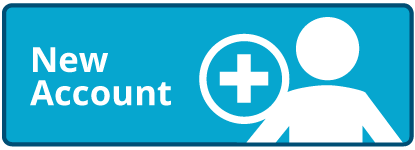# Multiplying 2-Digit by 1-Digit Numbers

Times up!
Times up! You may finish answering the current question and then proceed to your score!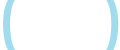Sorry, you have used all of your available hints for this lesson.
Practice Limit Reached

You've reached your daily practice limit of 12 questions.

Award InformationAwarded toQuestion HintChoose Family Member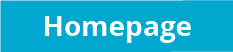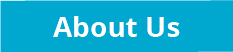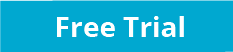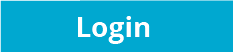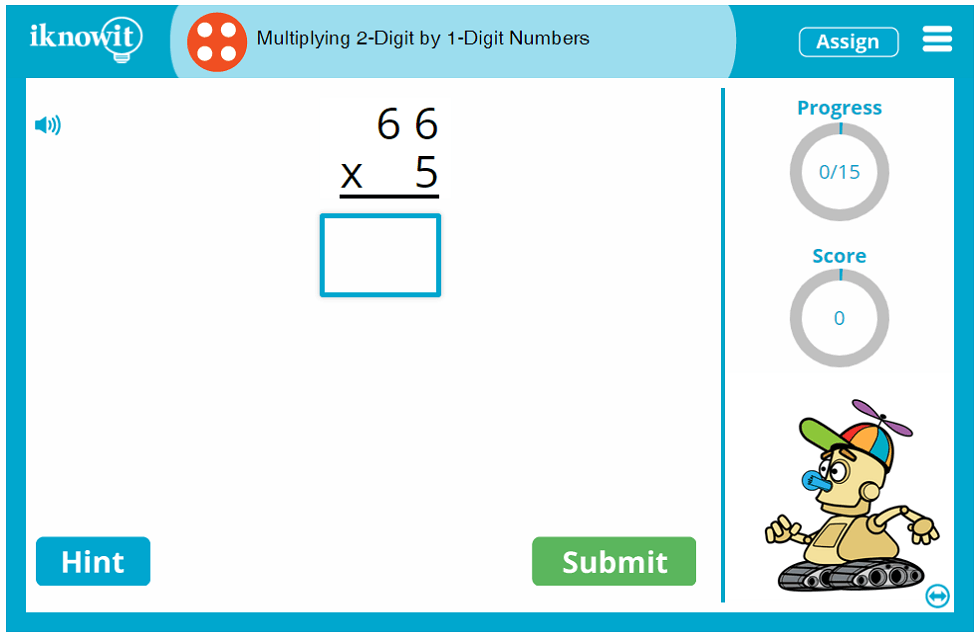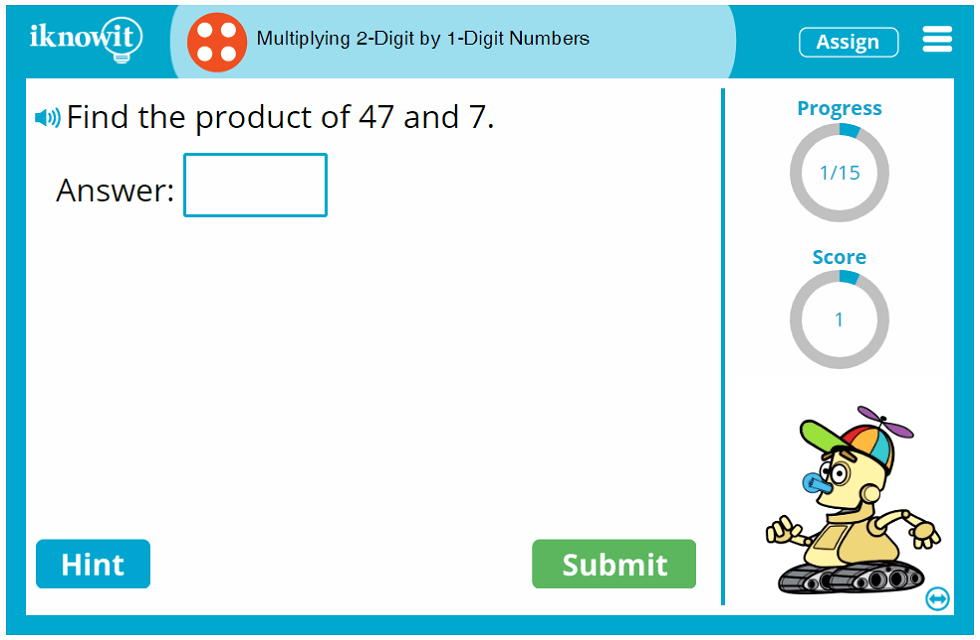## Online Math Activity - Multiplying 2-Digit by 1-Digit Numbers

Searching for a fun, educational online math activity to help your students practice basic multiplication skills? Try out this fun math lesson from "I Know It" featuring multiplication with 2-digit and 1-digit numbers. This online math game is presented at a fourth-grade difficulty level. Your students will gain proficiency in basic multiplication skills in this straightforward, yet challenging math lesson.

Students will answer a variety of vertical multiplication problems, horizontal multiplication problems, and multiplication word problems in this fourth-grade math game. Students may be asked to find the product of 14 and 6, 29 and 2, or 78 and 5. Or they may be given a word problem like this one: "The bookshelf in Mrs. Kinderman's classroom has three shelves. If she can fit 36 chapter books on each shelf, how many books are on the bookshelf in all?" If students need a little extra help solving a multiplication problem, they can click on the hint button, which will show the first step to solving the multiplication problem to help kids get started. When students answer a question incorrectly, a detailed explanation page will show the correct answer with an easy-to-follow explanation, helping children to learn from their mistakes as they move through the lesson.

In this fourth-grade multiplication activity, as well as all math lessons on the "I Know It" website, several handy features help students make the most of their math practice session. A progress-tracker in the upper-right corner of the practice screen lets students see how many questions they have answered so far in the lesson, while a score-tracker beneath that shows them how many points they have earned for right answers. A speaker icon indicates the read-aloud feature; students can click this button to have the question read out loud to them in a clear voice. (This is a great option for ESL/ELL students and for students who prefer auditory learning.) Each tool in our online math lessons is designed with your elementary students' success in mind!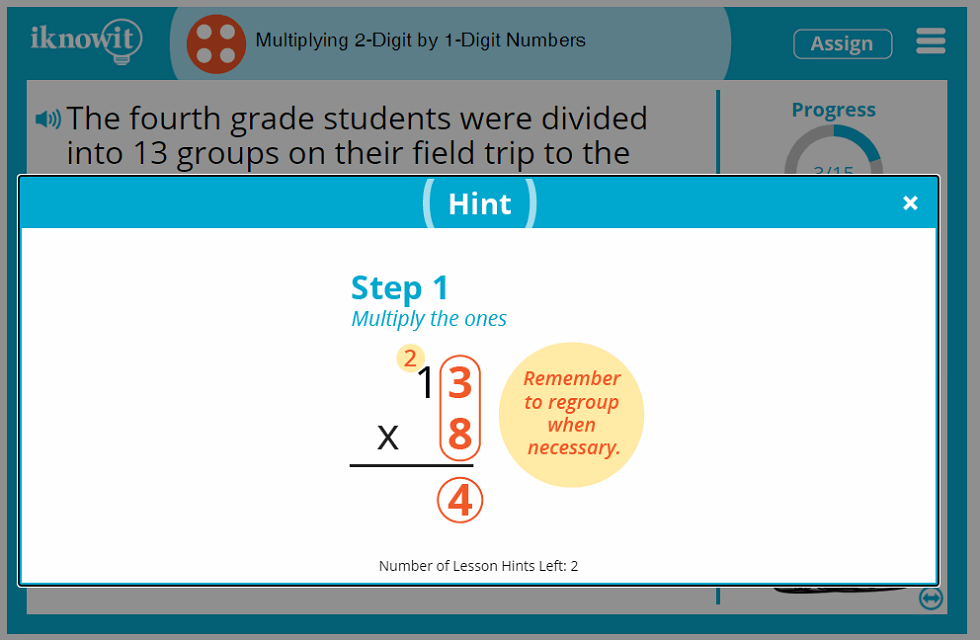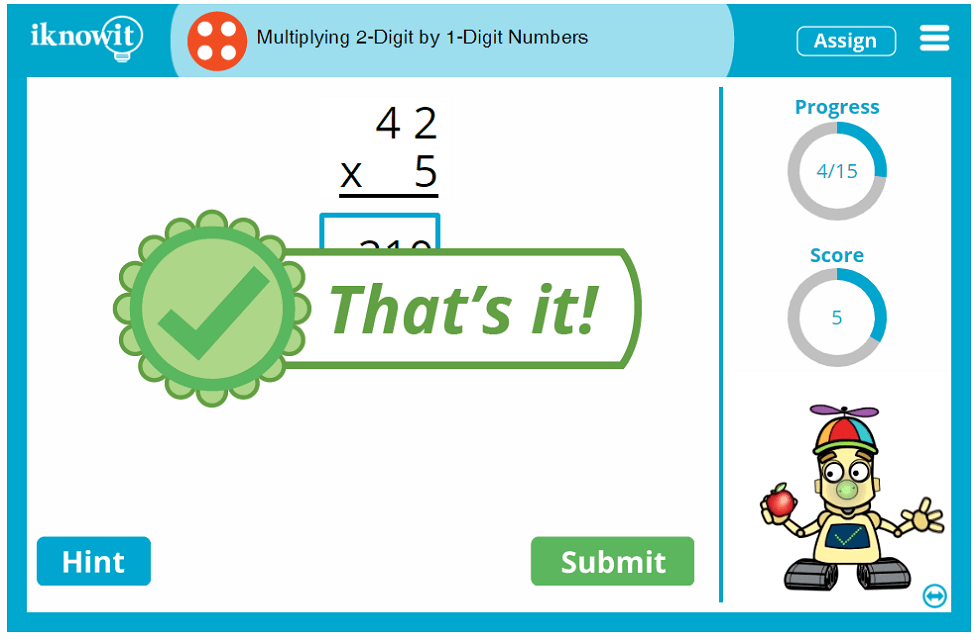## 'I Know It' Is the Right Choice for Teachers and Students Alike

Both teachers and students love using the 'I Know It' math practice program! Elementary teachers and homeschool educators appreciate the wide variety of math content we offer for kindergarten through fifth grade students. Each of our expertly written math lessons meets at least one Common Core Standard. You can find all of our math activities arranged on our website by grade level and topic, making it easy to find and assign math lessons in just a few clicks. Students, too, love practicing their math skills using the fun, interactive "I Know It" program. Adorable animated characters cheer students on in each math lesson, and plenty of positive messages encourage kids to "Keep going!" even when they make mistakes. Plus, the opportunity to earn math awards for mastering different skills makes math practice even more fun—especially when you can celebrate your students' math milestones together as a class!

We hope you and your fourth-grade class will have fun practicing multiplication with 2-digit and 1-digit numbers in this interactive math activity! Be sure to check out the hundreds of other 4th grade math lessons we have available on our website as well.

## Free Trial and Membership Options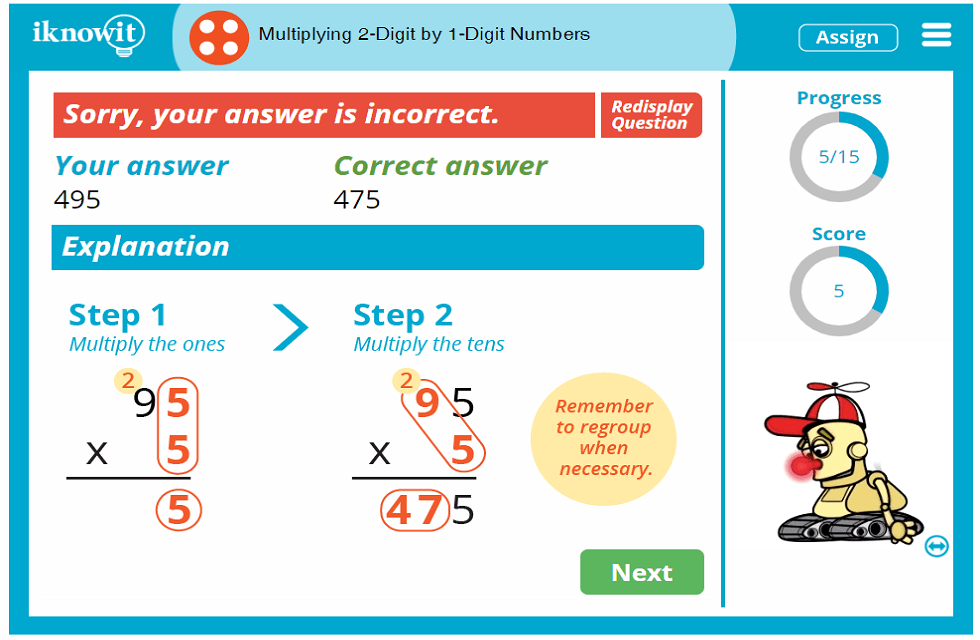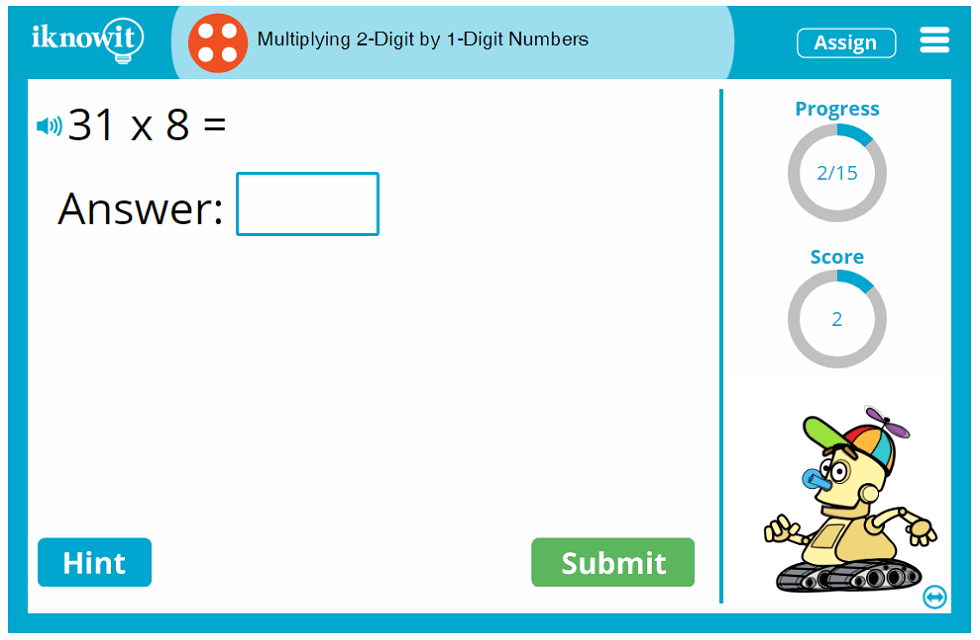## Level

This online math lesson is classified as Level D. It may be ideal for a fourth-grade class.

## Common Core Standard

4.NBT.5
Numbers and Operations in Base Ten

Students will use their understanding of place value and the properties of operations to perform multi-digit arithmetic.

## You might also be interested in...

Multiplying 3-Digit by 1-Digit Numbers (Level D)
In this fourth grade-level math lesson, students will practice multiplying 3-digit numbers by 1-digit numbers. Students will solve vertical multiplication problems, horizontal multiplication problems, and multiplication word problems.

Multiplying 4-Digit by 1-Digit Numbers (Level D)
In this math lesson geared toward fourth-grade, students will practice multiplying 4-digit numbers by 1-digit numbers. Students will solve horizontal multiplication problems, vertical multiplication problems, and multiplication word problems.